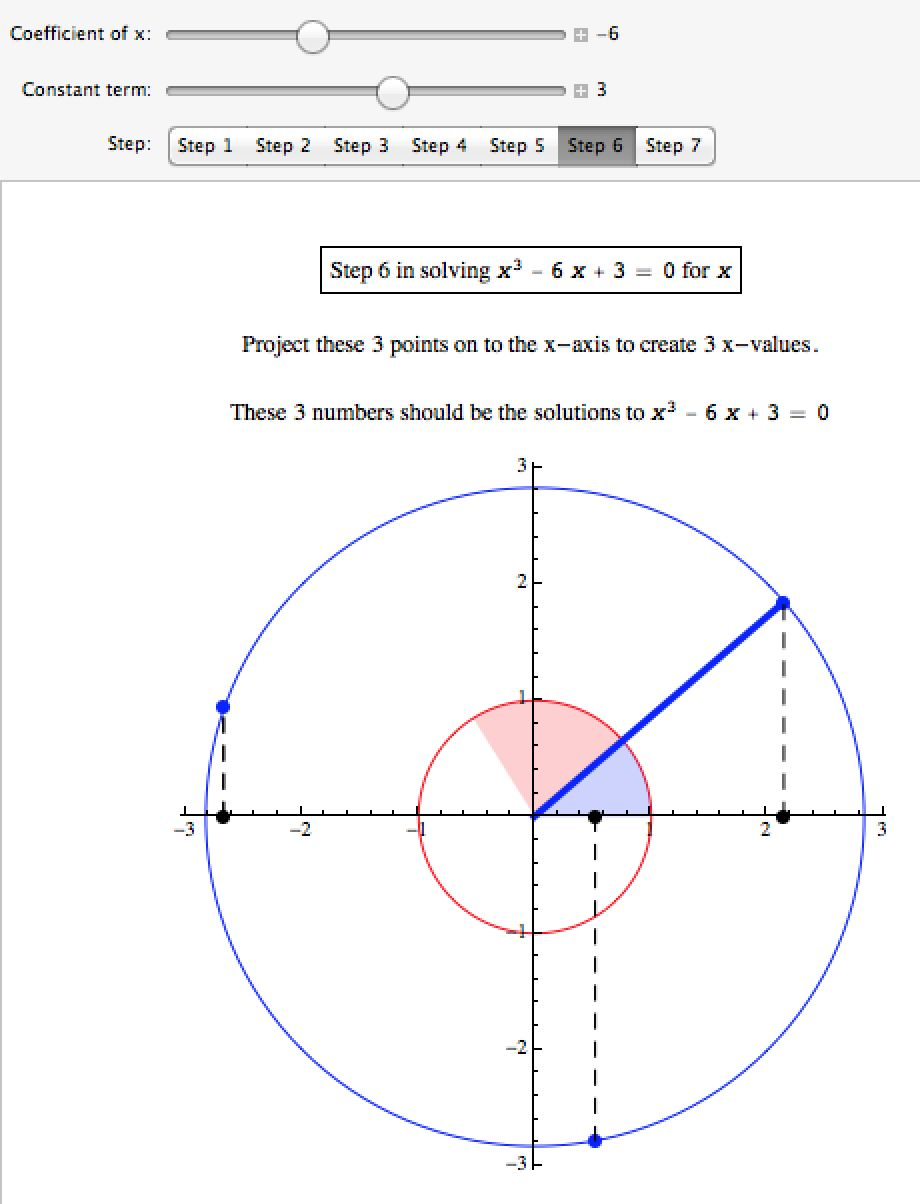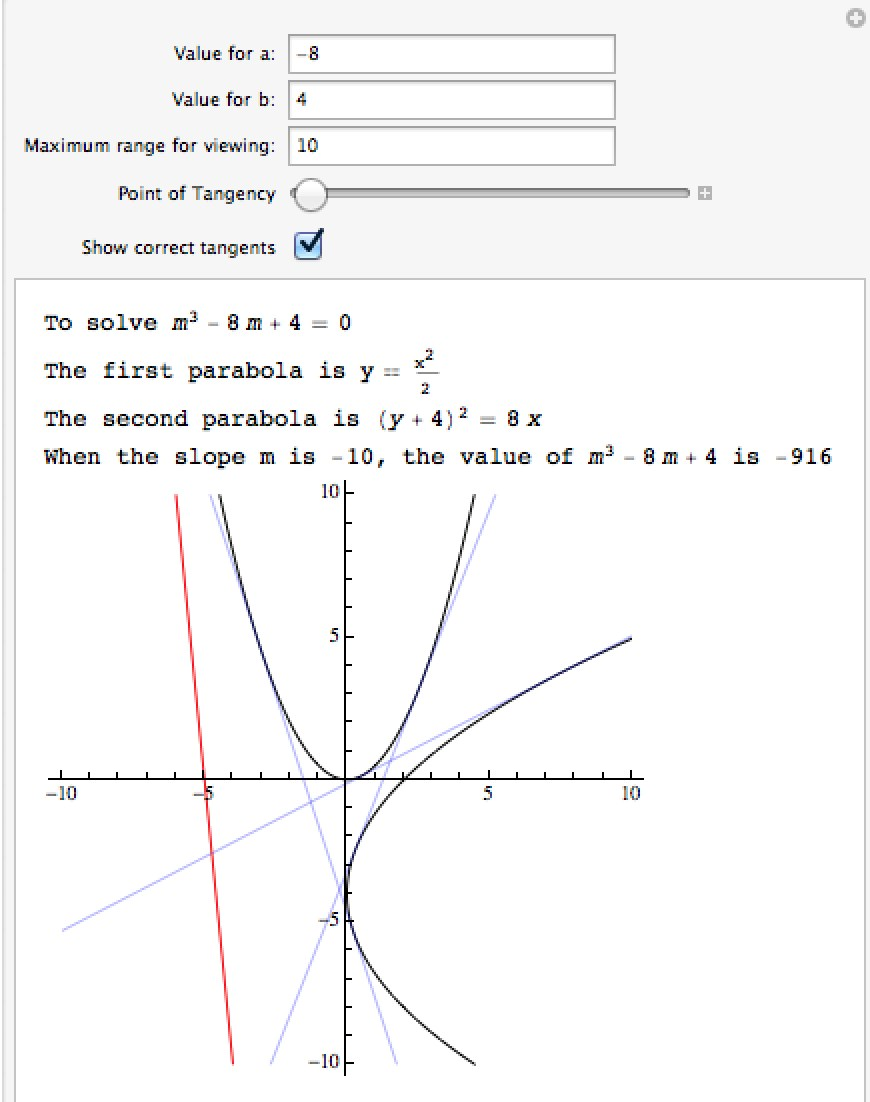# Mathematica Notebooks for Standard Algebra

Important Note: The links for the notebooks open a new window or tab with a Google Drive page – the current settings for our homepages won’t allow me to host mathematica notebooks locally.

## Slope and Slope Intercept

This notebook contains two simple manipulations to illustrate the idea of the slope between two points and the slope-intercept form of a line.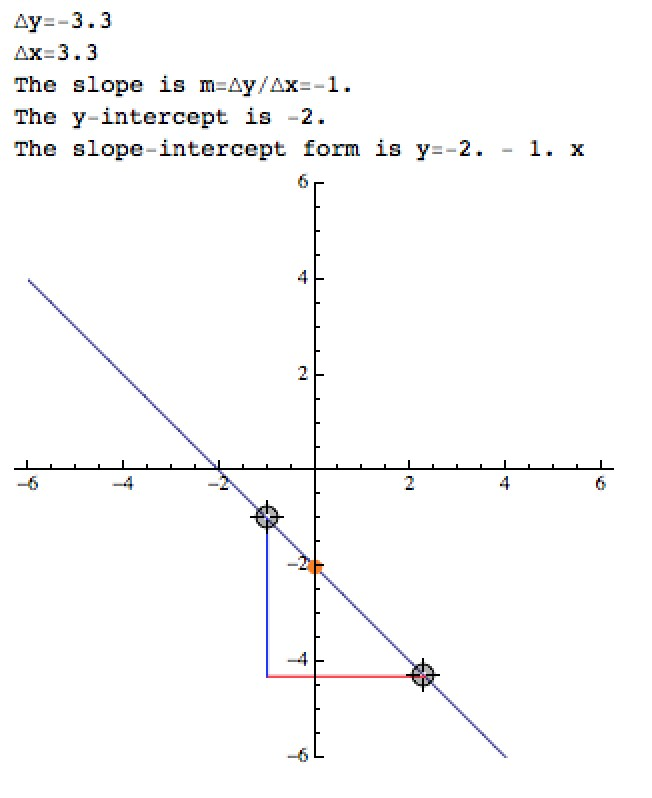## Roots of Polynomials

This notebook has a manipulation which displays the complex roots of a polynomial graphically in the complex plane.  By changing the degree and selecting “Random Polynomial”, you can see the Fundamental Theorem of Algebra (a polynomial of degree n has n roots) and the Conjugate Theorem (if p(x) is a polynomial with real coefficients and a+bi is a root, so is a-bi).  Real roots are blue, nonreal roots in green.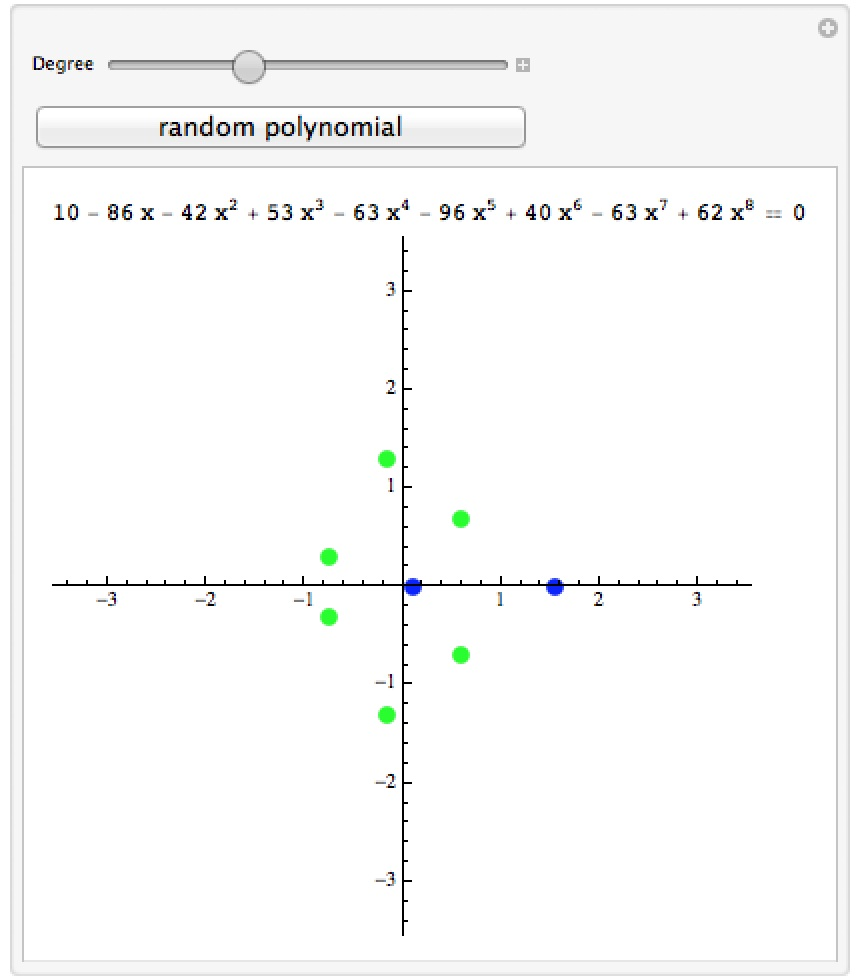## Multiplying Polynomials

This notebook demonstrates how to multiply two polynomials step by step.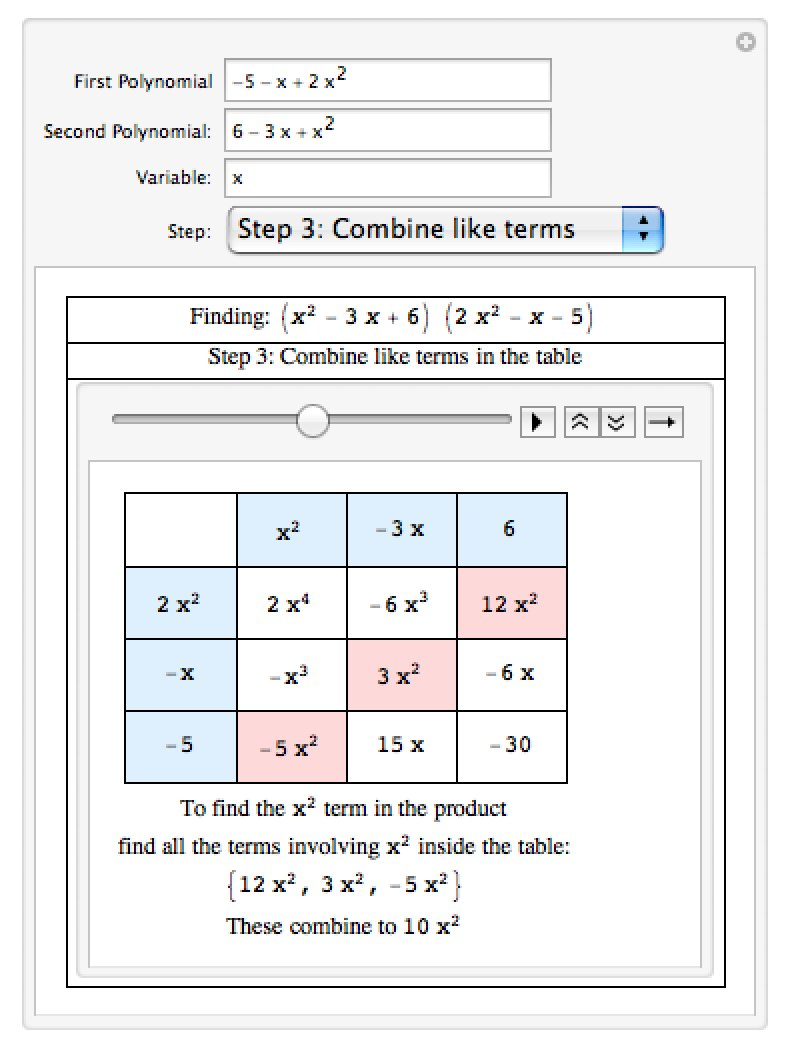## Solving Cubic Equations by Trisections

This notebook demonstrates the steps to solving a cubic equation geometrically by trisecting a particular angle.  You can find a version of this on the Wolfram Demonstrations Project.